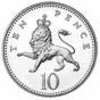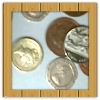#### You may also like### Coin Tossing Games

You and I play a game involving successive throws of a fair coin. Suppose I pick HH and you pick TH. The coin is thrown repeatedly until we see either two heads in a row (I win) or a tail followed by a head (you win). What is the probability that you win?### Win or Lose?

A gambler bets half the money in his pocket on the toss of a coin, winning an equal amount for a head and losing his money if the result is a tail. After 2n plays he has won exactly n times. Has he more money than he started with?### Thank Your Lucky Stars

A counter is placed in the bottom right hand corner of a grid. You toss a coin and move the star according to the following rules: ... What is the probability that you end up in the top left-hand corner of the grid?

# Mathsland National Lottery

##### Age 14 to 16Challenge Level

We hope that you enjoyed exploring this problem.

Here are some of the solutions which we have previously received from students:

Derin from Woodhouse College gave a clear presentation of his reasoning:

At the first draw, when there are 6 balls in the bag, you want one of your 3 numbers to be picked, so the chance of that happening is $\frac{3}{6}$ (or $\frac{1}{2}$).

For your second ball to be chosen, when there are five balls left in the bag and you want one of your 2 remaining numbers to be picked, the chance of that happening is $\frac{2}{5}$.

For the last ball to be chosen, when there are four balls left in the bag and you want your one remaining number to be picked, the chance of that happening is $\frac{1}{4}$.

In order for all three of your balls to be picked sequentially, you must multiply the probabilities of each being chosen on their own, i.e:$\frac{3}{6}\times\frac{2}{5}\times\frac{1}{4}= \frac{1}{20}$ therefore you have a one-in-twenty (or 0.05) chance of winning.

Ayden from Melbourn Village College calculated the solution to the rest of the problem. He also noticed that the chances of picking two balls out of six and four balls out of six is the same.

If you had a 2 out of 6 ball lottery it would increase your chances of winning:
$\frac{2}{6}\times\frac{1}{5}= \frac{1}{15}$

Drawing 4 balls instead of 2 will keep the chance of winning the same:
$\frac{4}{6}\times\frac{3}{5}\times\frac{2}{4}\times\frac{1}{3}= \frac{1}{15}$

Having a 1-ball lottery will dramatically increase your chance of winning to $\frac{1}{6}$.

Having a 5 ball lottery will give you the same chance of winning:
$\frac{5}{6}\times\frac{4}{5}\times\frac{3}{4}\times\frac{2}{3}\times\frac{1}{2}= \frac{1}{6}$

To minimise the chance of winning a 10-ball lottery, the organisers should require participants to pick 5 balls.

Phil from Wilson's School provided the explanation:

If the Mathsland Lottery changes to a 10-ball lottery and wants to minimise the chance of winning, then they should ask participants to pick 5 balls correctly to win. This is because if you look at the six-ball lottery the chance of winning was its lowest when three balls were needed to be picked correctly. This is half of three, and on either side of 3 balls, 2 and 4, the chance of winning went back up.

When 5 out of ten must be picked, the chance of winning is
$\frac{5}{10}\times\frac{4}{9}\times\frac{3}{8}\times\frac{2}{7}\times\frac{1}{6}= \frac{24}{6048}=\frac{1}{252}$
which is smaller than when 4 balls have to be picked:
$\frac{4}{10}\times\frac{3}{9}\times\frac{2}{8}\times\frac{1}{7}=\frac{1}{210}$
and when 6 balls have to be picked:
$\frac{6}{10}\times\frac{5}{9}\times\frac{4}{8}\times\frac{3}{7}\times\frac{2}{6}\times\frac{1}{5}=\frac{1}{210}$
As we get further away from picking 5, the probabilities keep increasing:
When 3 balls have to be picked:
$\frac{3}{10}\times\frac{2}{9}\times\frac{1}{8}=\frac{1}{120}$
and when 8 balls have to be picked:
$\frac{8}{10}\times\frac{7}{9}\times\frac{6}{8}\times\frac{5}{7}\times\frac{4}{6}\times\frac{3}{5}\times\frac{2}{4}\times\frac{1}{3}=\frac{1}{45}$

Therefore if the Mathsland lottery is organising a ten-ball lottery, participants should be ask to predict 5 balls, since this results in the lowest winning chances.

It is worth pointing out that picking 2 balls out of 6 balls is essentially the same as picking 4 balls out of 6 balls, since in both cases 2 balls need to be identified and separated. The two processes are equivalent, so the probabilities match. The same goes for picking 1 out of 6 and picking 5 out of 6, etc.

The chance of winning the UK National lottery, in which participants choose 6 numbers from 49, is:

$\frac{6}{49}\times\frac{5}{48}\times\frac{4}{47}\times\frac{3}{46}\times\frac{2}{45}\times\frac{1}{44}=\frac{1}{13,983,816}$ or approximately 1 in 14 million.

Well done to everyone!